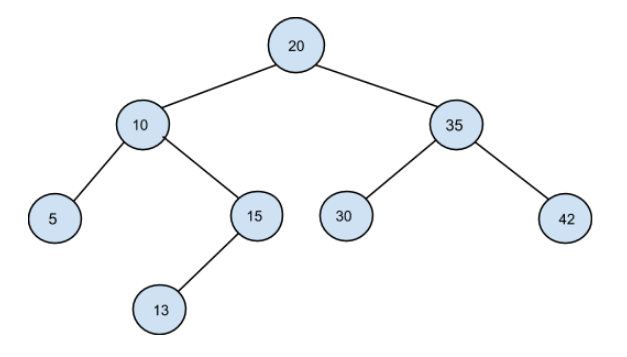# Preorder traversal of a BST

Posted: 24 Nov, 2020
Difficulty: Moderate

## PROBLEM STATEMENT

#### A binary search tree (BST) is a binary tree data structure that has the following properties:

``````• The left subtree of a node contains only nodes with data less than the node’s data.
• The right subtree of a node contains only nodes with data greater than the node’s data.
• Both the left and right subtrees must also be binary search trees.
``````

#### Note:

``````It is guaranteed that a BST can be always constructed from the given preorder traversal. Hence, the answer will always exist.
``````
##### Example:
``````From PREORDER = [20, 10, 5, 15, 13, 35, 30, 42] , the following BST can be constructed:
``````##### Input Format:
``````The first line contains an integer 'T' which denotes the number of test cases or queries to be run. Then the test cases follow.

The first line of each test case contains a single integer 'N' denoting the number of nodes in BST.

The second line of each test case contains 'N' single space-separated integers representing the preorder traversal of BST.
``````
##### Output Format:
``````Print the inorder traversal of the constructed BST where all nodes are printed in a single-space separated manner.

Output for every test case will be printed in a separate line.

Note:
You don't need to print anything. It has already been taken care of. Just implement the function.
``````
##### Constraints :
``````1 <= T <= 100
1 <= N <= 5000
0 <= data <= 10^5

Where 'data' denotes data contained in the nodes of the binary search tree.

Time Limit: 1 sec
``````Approach 1

We have a simple Brute Force solution for this problem. We will traverse the PREORDER array, find the left subtree and right subtree and then recursively construct the tree as follows-

• The first element of the preorder traversal corresponds to the root of BST.
• We will find the index ‘SPLIT’ of the first element in the given traversal which is greater than the root node i.e PREORDER[‘SPLIT’] > PREORDER['START'].
• We will split the given array to the left subarray ['START' + 1 : ‘SPLIT’ - 1] and right subarray [‘SPLIT’: ‘END’] corresponding to the left subtree and right subtree, respectively.
• We then recursively repeat the above steps for both subtrees to construct the complete tree.